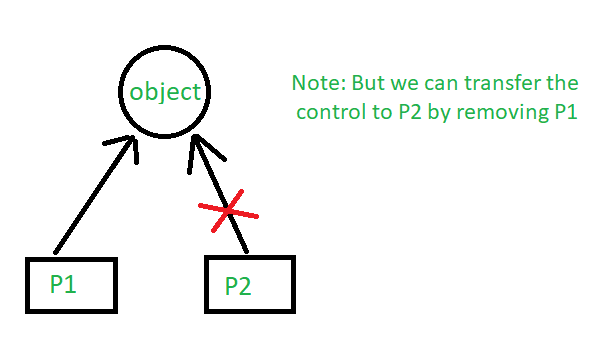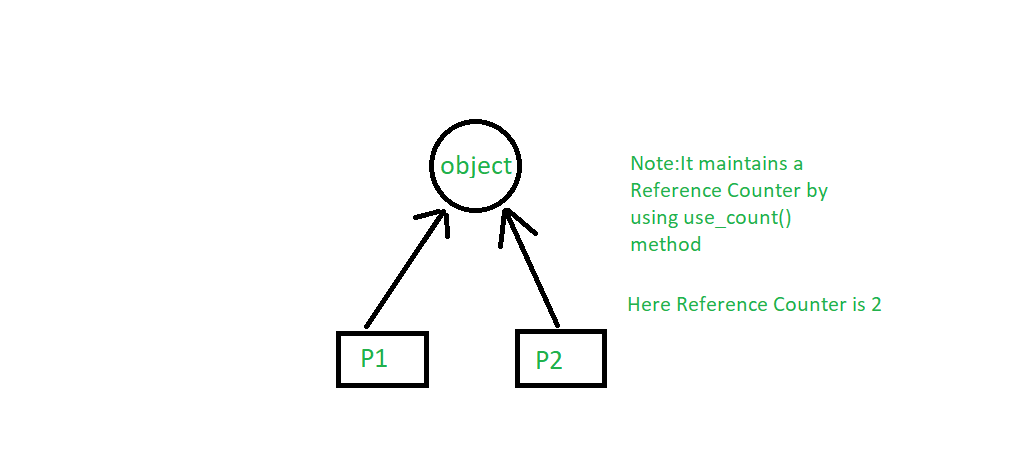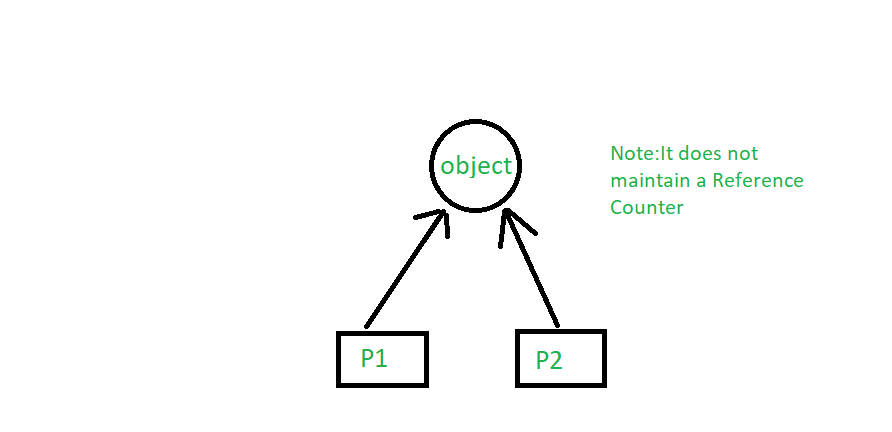Related Articles
Introduction of Smart Pointers in C++ and It’s Types
• Difficulty Level : Medium
• Last Updated : 01 Apr, 2020

Things to learn in this article:

1. Importance of pointer in C/C++.
2. Problems with normal pointer.
3. Why smart pointers introduce.
4. Types of smart pointer.

Importance of pointer in C/C++:
Pointers are used for accessing the resources which are external to the program like heap memory. So for accessing the heap memory if anything is created inside heap memory Pointers are used.

Problem with normal pointer:
Let’s understand what’s the main problem with the normal pointer by taking a small C++ program using this illustration.

 `#include ``using` `namespace` `std;`` ` `class` `Rectangle {``private``:``    ``int` `length;``    ``int` `breadth;``};`` ` `void` `fun()``{``    ``// By taking a pointer p and``    ``// dynamically creating object``    ``// of class rectangle``    ``Rectangle* p = ``new` `Rectangle();``}`` ` `int` `main()``{``    ``// Infinite Loop``    ``while` `(1) {``        ``fun();``    ``}``}`

So what happens is it’ll have a pointer ‘p’ and this will be pointing to an object of type rectangle which will have length and breadth. Once the function ends this ‘p’ is deleted because p is a local variable to the function which will end but a new rectangle that is allocated inside heap that will not be deallocated. And it will return it will come back as its infinite loop again it will call, so again new p is created then again a new object is created for rectangle same length and breadth. So then what about the previous object, it’ll not be deleted, again extra new object, it’ll also not deleted. So every time it will create an object but not it’s deleting so this is causing leakage of memory from the heap memory. Like for memory for length and breadth is unused though it’s allocated but not in use. So slowly the entire heap memory may become unused because it’s infinite. So at one stage because of a lack of heap memory, the program will crash. So at the last of the fun() we should use ‘delete p’ if we do not mention this, this will cause a very severe problem. so because of the laziness or carelessness of the programmer this type of problem may arise. So to help the programmer C++ 11 takes responsibility and introduces smart pointers.

Introduction of Smart Pointers
The problem with heap memory is that when you don’t need it you must deallocate itself. So mostly the programmers are too lazy in writing the code for deallocation of objects and that causes severe problem like memory leak which will cause the program to crash. So the languages like Java, C#, .Net Framework they provide a garbage collection mechanism to deallocate the object which is not in use. So in C++ 11, it introduces smart pointers that automatically manage memory and they will deallocate the object when they are not in use when the pointer is going out of scope automatically it’ll deallocate the memory.

Consider the following simple C++ code with normal pointers.

 `MyClass* ptr = ``new` `MyClass();``ptr->doSomething();``// We must do delete(ptr) to avoid memory leak`

Using Smart Pointers, we can make pointers to work in a way that we don’t need to explicitly call delete. A smart pointer is a wrapper class over a pointer with an operator like * and -> overloaded. The objects of smart pointer class look like a pointer but can do many things that a normal pointer can’t like automatic destruction (yes, we don’t have to explicitly use delete), reference counting and more.
The idea is to take a class with a pointer, destructor and overloaded operators like * and ->. Since the destructor is automatically called when an object goes out of scope, the dynamically allocated memory would automatically be deleted (or reference count can be decremented). Consider the following simple smart ptr class.

 `#include ``using` `namespace` `std;`` ` `class` `SmartPtr {``    ``int``* ptr; ``// Actual pointer``public``:``    ``// Constructor: Refer https:// www.geeksforgeeks.org/g-fact-93/``    ``// for use of explicit keyword``    ``explicit` `SmartPtr(``int``* p = NULL) { ptr = p; }`` ` `    ``// Destructor``    ``~SmartPtr() { ``delete` `(ptr); }`` ` `    ``// Overloading dereferencing operator``    ``int``& operator*() { ``return` `*ptr; }``};`` ` `int` `main()``{``    ``SmartPtr ptr(``new` `int``());``    ``*ptr = 20;``    ``cout << *ptr;`` ` `    ``// We don't need to call delete ptr: when the object``    ``// ptr goes out of scope, the destructor for it is automatically``    ``// called and destructor does delete ptr.`` ` `    ``return` `0;``}`
Output:
```20
```

Writing one smart pointer class that works for all types.
Yes, we can use templates to write a generic smart pointer class. Following C++ code demonstrates the same.

 `#include ``using` `namespace` `std;`` ` `// A generic smart pointer class``template` `<``class` `T>``class` `SmartPtr {``    ``T* ptr; ``// Actual pointer``public``:``    ``// Constructor``    ``explicit` `SmartPtr(T* p = NULL) { ptr = p; }`` ` `    ``// Destructor``    ``~SmartPtr() { ``delete` `(ptr); }`` ` `    ``// Overloading dereferncing operator``    ``T& operator*() { ``return` `*ptr; }`` ` `    ``// Overloading arrow operator so that``    ``// members of T can be accessed``    ``// like a pointer (useful if T represents``    ``// a class or struct or union type)``    ``T* operator->() { ``return` `ptr; }``};`` ` `int` `main()``{``    ``SmartPtr<``int``> ptr(``new` `int``());``    ``*ptr = 20;``    ``cout << *ptr;``    ``return` `0;``}`
Output:
```20
```

Note: Smart pointers are also useful in the management of resources, such as file handles or network sockets.

Types of Smart Pointer:

1. unique_ptr
If you are using a unique pointer then if one object is created and pointer P1 is pointing to this one them only one pointer can point this one at one time. So we can’t share with another pointer, but we can transfer the control to P2 by removing P1.`#include ``using` `namespace` `std;``#include `` ` `class` `Rectangle {``    ``int` `length;``    ``int` `breadth;`` ` `public``:``    ``Rectangle(``int` `l, ``int` `b)``    ``{``        ``length = l;``        ``breadth = b;``    ``}`` ` `    ``int` `area()``    ``{``        ``return` `length * breadth;``    ``}``};`` ` `int` `main()``{`` ` `    ``unique_ptr P1(``new` `Rectangle(10, 5));``    ``cout << P1->area() << endl; ``// This'll print 50`` ` `    ``// unique_ptr P2(P1);`` ` `    ``unique_ptr P2;``    ``P2 = move(P1);`` ` `    ``// This'll print 50``    ``cout << P2->area() << endl;`` ` `    ``// cout<area()<
Output:
```50
50
```
2. shared_ptr
If you are using shared_ptr then more than one pointer can point to this one object at a time and it’ll maintain a Reference Counter using use_count() method.`#include ``using` `namespace` `std;``#include `` ` `class` `Rectangle {``    ``int` `length;``    ``int` `breadth;`` ` `public``:``    ``Rectangle(``int` `l, ``int` `b)``    ``{``        ``length = l;``        ``breadth = b;``    ``}`` ` `    ``int` `area()``    ``{``        ``return` `length * breadth;``    ``}``};`` ` `int` `main()``{`` ` `    ``shared_ptr P1(``new` `Rectangle(10, 5));``    ``// This'll print 50``    ``cout << P1->area() << endl;`` ` `    ``shared_ptr P2;``    ``P2 = P1;`` ` `    ``// This'll print 50``    ``cout << P2->area() << endl;`` ` `    ``// This'll now not give an error,``    ``cout << P1->area() << endl;`` ` `    ``// This'll also print 50 now``    ``// This'll print 2 as Reference Counter is 2``    ``cout << P1.use_count() << endl;``    ``return` `0;``}`
Output:
```50
50
50
2
```
3. weak_ptr
It’s much more similar to shared_ptr except it’ll not maintain a Reference Counter.In this case, a pointer will not have a strong hold on the object. The reason is if suppose pointers are holding the object and requesting for other objects then they may form a Deadlock.C++ libraries provide implementations of smart pointers in the form of auto_ptr, unique_ptr, shared_ptr and weak_ptr

References:
http://en.wikipedia.org/wiki/Smart_pointer

This article is improved by AmiyaRanjanRout.Please write comments if you find anything incorrect, or you want to share more information about the topic discussed above.

Want to learn from the best curated videos and practice problems, check out the C++ Foundation Course for Basic to Advanced C++ and C++ STL Course for foundation plus STL.  To complete your preparation from learning a language to DS Algo and many more,  please refer Complete Interview Preparation Course.
My Personal Notes arrow_drop_up